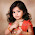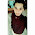## Sunday, February 22, 2015

### Introduction to tries through Facebook Hacker Cup 2015 Round 1 - Autocomplete

In the last week's post I discussed the solution to the problem "Homework" from Facebook Hacker Cup 2015 Round 1. This post is about the problem "Autocomplete" from the same round.

I will re-state the problem in fewer lines. You have a new phone which has an auto-complete feature. But, it will complete your word only if there is there is no ambiguity on how the word should be completed. Formally, it will complete a word iff there is exactly one way to complete the word. It so happens that the phone's dictionary is empty by default. So, for the auto-complete feature to work, before typing a word you need to add that word to the dictionary. You need to send a message containing $$N$$ words. What is the minimum number of characters must you type to send all $$N$$ words? (Note that the typing involved in adding a word to the dictionary is not counted.) You need to solve $$T$$ instances of this problem.

Here are the constraints:
• $$1 \le T \le 100$$
• $$1 \le N \le 100,000$$

The solution idea is pretty straightforward. For any word $$w$$, the minimum characters that have to be typed is equal to length of the shortest prefix $$p$$ of $$w$$ such that there is no word in the dictionary that has a prefix $$p$$. It turns out that there is a very elegant solution using tries.

### What is a trie?

A trie is a data structure used to store string keys for fast look-up. There are many resources online that have explained tries better than I can. So, I am just gonna give an brief overview here and point you to useful online resources. Later, I will how tries can be implemented easily in a programming contest environment.

Typically, a trie looks like this.

Notice that every node contains 26 pointers corresponding to each letter in the English alphabet. The topmost node in the above figure is called the root node. We see that only the pointers corresponding to M, P and T are depicted. We can assume the other pointers to be NULL i.e. there are no words starting with characters other than M, P and T. When we follow the M pointer we reach another node which has pointers only for A and E. This means that there are words in the trie with prefixes MA and ME. If we continue following the E branch we reach a node with a Δ symbol. The Δ symbol signifies that a word ends there. This means that the word $$\text{MENDEL}$$ is present in the trie. This node also has a pointer corresponding to E. This is not the beginning of a new word. It is just a continuation of $$\text{MENDEL}$$. Following the pointers leads us to the word $$\text{MENDELEEV}$$. Similarly, the words $$\text{MAXWELL}$$, $$\text{PASTEUR}$$, $$\text{PAVLOV}$$, $$\text{PEANO}$$, $$\text{POINCARE}$$, $$\text{POISSON}$$ and $$\text{TURING}$$ are present in the trie.

If you have visited above links you would have noticed that tries have complicated and messy implementation involving dynamic memory allocation. This is unsuitable for programming contests. Here is a more contest-friendly trie implementation.
int a[N];
char s[N];
int n = 1;

scanf("%s", s);
int t = 1;
for (int i = 0; s[i]; ++i) {
int p = s[i] – 'a'
if (a[t][p] == 0) {
n++;
a[t][p] = n;
}
t = a[t][p];
}
The above shows how to implement the insert operation in a simple way. All we are doing here is simulating dynamic memory allocation in place of actually using it. In the above code a[][] denotes the trie and n denotes the index of the next available node. If you have read the GeeksforGeeks article it should not be very difficult to understand how the above code works. Can you figure out how to write code for the look-up operation? Here is my implementation:
bool isPresent(char *s) {
int t = 1;
for (int i = 0; s[i]; ++i) {
int p = s[i] - 'a';
if (a[t][p] == 0) return false;
else t = a[t][p];
}
return true;
}

Know that we know how tries work and how to implement them, let's solve the original problem. As I pointed out earlier, we need to find, for every word, how much of the word has to be typed such that the rest of the word can be uniquely identified. This is the same as finding the shortest prefix of the word that is not present in the trie. Let's follow this algorithm:
for every word w do:
add it to the trie in the following way:
during adding count the length of the longest prefix that is already present
call this length L
repeat

This algorithm leads to a simple implementation. You don't even need to write a separate function for look-up. Here is my implementation:
const int N = 1000050;

int a[N];
char s[N];

int main() {
int t;
scanf("%d", &t);
long long ans;
for (int tt = 1; tt <= t; ++tt) {
printf("Case #%d: ", tt);
int nn;
scanf("%d", &nn);
ans = 0;
int n = 1;
for (int i = 0; i < nn; ++i) {
scanf("%s", s);
int t = 1, ct = 0;
bool end = false;
for (int j = 0; s[j]; ++j) {
int p = s[j] - 'a';
if (!end) ct++;
if (a[t][p] == 0) {
n++;
a[t][p] = n;
end = true;
}
t = a[t][p];
}
ans += ct;
}
printf("%lld\n", ans);
memset(a, 0, sizeof(a));
}
return 0;
}

1.Thanks for sharing this informative blog.. Your information related to hacking is really useful for me. Keep posting..

Regards..
Hacking Course in Chennai

1.A IEEE project is an interrelated arrangement of exercises, having a positive beginning and end point and bringing about an interesting result in Engineering Colleges for a particular asset assignment working under a triple limitation - time, cost and execution. Final Year Project Domains for CSE In Engineering Colleges, final year IEEE Project Management requires the utilization of abilities and information to arrange, plan, plan, direct, control, screen, and assess a final year project for cse. The utilization of Project Management to accomplish authoritative objectives has expanded quickly and many engineering colleges have reacted with final year IEEE projects Project Centers in Chennai for CSE to help students in learning these remarkable abilities.

Spring Framework has already made serious inroads as an integrated technology stack for building user-facing applications. Spring Framework Corporate TRaining the authors explore the idea of using Java in Big Data platforms.
Specifically, Spring Framework provides various tasks are geared around preparing data for further analysis and visualization. Spring Training in Chennai

2.As a rule of thumb, talk about special occasions in the past tense rather than present or future. For example, don't announce to the world via Facebook that you're now leaving to house for a night of dinner, movies, and dancing, as people will then know you'll be away from your house for the next 5 hours.
3.4.5.